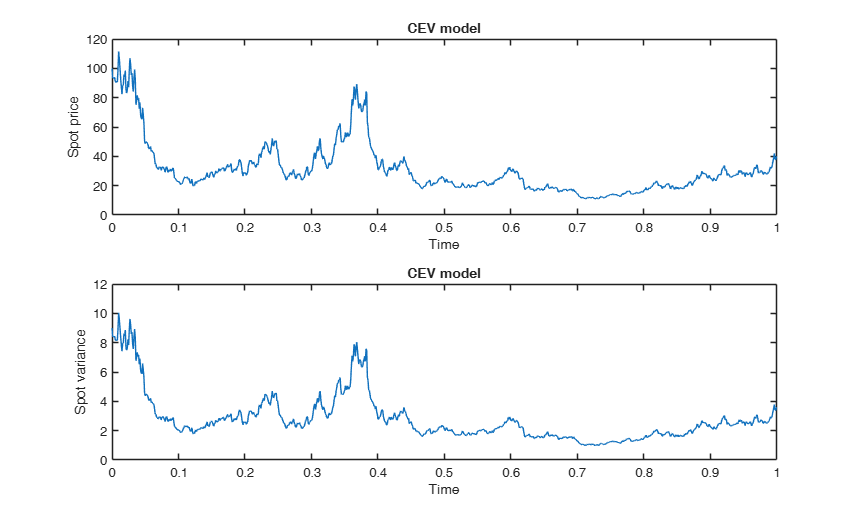CEVmodel

CEVmodel computes price and instantaneous variance processes from the CEV model

Description

CEVmodel computes price and instantaneous variance for the Constant Elasticity of Variance model [S. Beckers, The Journal of Finance, Vol. 35, No. 3, 1980] via Euler method

S =CEVmodel(t, x) Example of call of CEVmodel providing only price values.

[S, A] =CEVmodel(___) Example of call of CEVmodel providing both price and variance values.

Examples

expand allExample of call of CEVmodel providing only price values.

Generates spot prices for the CEV model at times t.

n=1000; dt=1/n;
t=0:dt:1; % discrete time grid
x=100; % initial price value
S=CEVmodel(t,x); % spot prices
plot(t,S)
xlabel('Time')
ylabel('Spot price')
title('CEV model')Example of call of CEVmodel providing both price and variance values.

Generates price and instantaneous variance values for the CEV model at times t.

n=1000; dt=1/n;
t=0:dt:1; % discrete time grid
x=100; % initial price value
[S,A]=CEVmodel(t,x); % spot prices and variance
subplot(2,1,1)
plot(t,S)
xlabel('Time')
ylabel('Spot price')
title('CEV model')
subplot(2,1,2)
plot(t,A)
xlabel('Time')
ylabel('Spot variance')
title('CEV model')Input Arguments

t — Discrete time grid. Vector.

Row or column vector.

Data Types: single| double

x — Initial price value. Scalar.

Data Types: single| double

Output Arguments

S —Spot prices. Vector

Column vector with the same length of t.

A —Spot variance values. Vector

Column vector with the same length of t.

The Constant Elasticity of Variance model [S. Beckers, The Journal of Finance, Vol. 35, No. 3, 1980] is given by the following stochastic differential equation $$\left\{\begin{array}{l} dS_t= \sigma \, S_t^{\delta} \, dW_t \\ S_0=x, \end{array}\right.$$ where $\sigma$ and $\delta$ are positive constants and $W$ is a Brownian motion on a filtered probability space. We assume $\sigma=0.3$ and $\delta=1.5$. The instanteneous variance is given by $$A_t=\sigma^2S_t^{2(\delta-1)}.$$